### HashMap Custom implementation in java - How HashMap works internally with diagrams and full program

You are here : Home / Core Java Tutorials / Data structures / Collection framework

Contents of page :
• 7.1) put method - worst Case complexity >
• 7.2) put method - best Case complexity >
• 7.3) get method - worst Case complexity >
• 7.4) get method - best Case complexity >

1) Custom HashMap in java >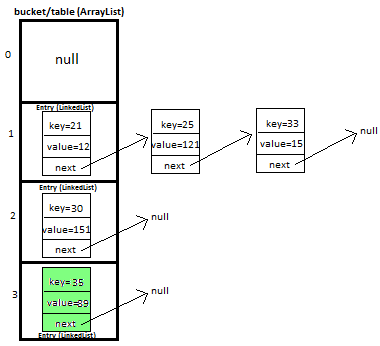In this tutorial we will learn how to create and implement own/custom HashMap in java with full working source code.

This is very important and trending topic in java. In this post i will be explaining HashMap custom implementation in lots of detail with diagrams which will help you in visualizing the HashMap implementation. This is must prepare topic for interview and from knowledge point of view as well.

I will be explaining how we will put and get key-value pair in HashMap by overriding-
>equals method - helps in checking equality of entry objects.
>hashCode method - helps in finding bucket’s index on which data will be stored.

We will maintain bucket (ArrayList) which will store Entry (LinkedList).

2) Entry<K,V>
We store key-value pair by using Entry<K,V>
Entry contains
• K key,
• V value and
• Entry<K,V> next  (i.e. next entry on that location of bucket).
 static class Entry {        K key;        V value;        Entry next;              public Entry(K key, V value, Entry next){            this.key = key;            this.value = value;            this.next = next;        }    }

3) Putting 5 key-value pairs in own/custom HashMap (step-by-step) in java>
I will explain you the whole concept of HashMap by putting 5 key-value pairs in HashMap.

Initially, we have bucket of capacity=4. (all indexes of bucket i.e. 0,1,2,3 are pointing to null)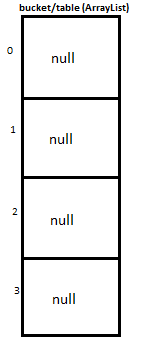Let’s put first key-value pair in HashMap-
Key=21, value=12
newEntry Object will be formed like this >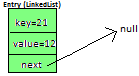We will calculate hash by using our hash(K key) method - in this case it returns
key/capacity= 21%4= 1.
So, 1 will be the index of bucket on which newEntry object will be stored.
We will go to 1st index as it is pointing to null we will put our newEntry object there.

At completion of this step, our HashMap will look like this-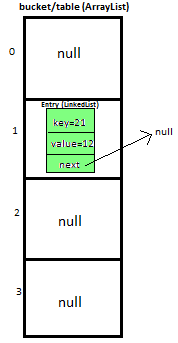Let’s put second key-value pair in HashMap-
Key=25, value=121
newEntry Object will be formed like this >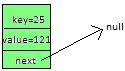We will calculate hash by using our hash(K key) method - in this case it returns
key/capacity= 25%4= 1.
So, 1 will be the index of bucket on which newEntry object will be stored.
We will go to 1st index, it contains entry with key=21, we will compare two keys(i.e. compare 21 with 25 by using equals method), as two keys are different we check whether entry with key=21’s next is null or not, if next is null we will put our newEntry object on next.

At completion of this step our HashMap will look like this-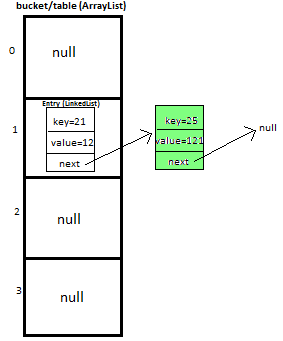Let’s put third key-value pair in HashMap-
Key=30, value=151
newEntry Object will be formed like this >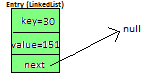We will calculate hash by using our hash(K key) method - in this case it returns
key/capacity= 30%4= 2.
So, 2 will be the index of bucket on which newEntry object will be stored.
We will go to 2nd  index as it is pointing to null we will put our newEntry object there.

At completion of this step, our HashMap will look like this-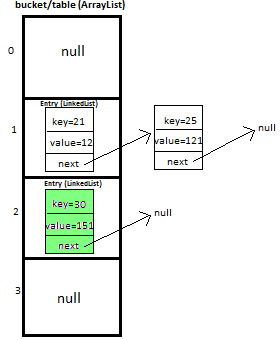Let’s put fourth key-value pair in HashMap-
Key=33, value=15
Entry Object will be formed like this >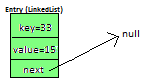We will calculate hash by using our hash(K key) method - in this case it returns
key/capacity= 33%4= 1,
So, 1 will be the index of bucket on which newEntry object will be stored.

We will go to 1st index -
>it contains entry with key=21, we will compare two keys (i.e. compare 21 with 33 by using equals method, as two keys are different,  proceed to next  of entry with key=21 (proceed only if next is not null).
>now, next contains entry with key=25, we will compare two keys (i.e. compare 25 with 33 by using equals method, as two keys are different,  now next of entry with key=25 is pointing to null so we won’t proceed further, we will put our newEntry object on next.

At completion of this step our HashMap will look like this-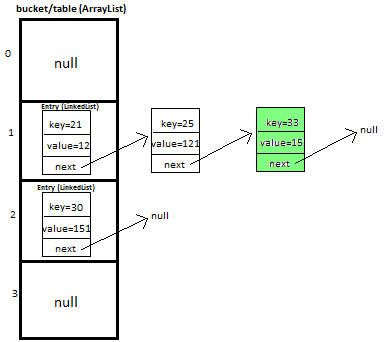Let’s put fifth key-value pair in HashMap-
Key=35, value=89

Repeat above mentioned steps.

At completion of this step our HashMap will look like this-4) Methods used in custom HashMap in java >
 public void put(K newKey, V data) -Method allows you put key-value pair in HashMap -If the map already contains a mapping for the key, the old value is replaced. -provide complete functionality how to override equals method. -provide complete functionality how to override hashCode method. public V get(K key) Method returns value corresponding to key. public boolean remove(K deleteKey) Method removes key-value pair from HashMapCustom. public void display() -Method displays all key-value pairs present in HashMapCustom., -insertion order is not guaranteed, for maintaining insertion order refer LinkedHashMapCustom. private int hash(K key) -Method implements hashing functionality, which helps in finding the appropriate bucket location to store our data. -This is very important method, as performance of HashMapCustom is very much dependent on  this method's implementation.

5) What will happen if map already contains mapping for key?
If the map already contains a mapping for the key, the old value is replaced.

6) Full Program/SourceCode for implementing custom HashMap in java >
 package com.ankit; /** * @author AnkitMittal, JavaMadeSoEasy.com * Copyright (c), AnkitMittal . All Contents are copyrighted and must not be * reproduced in any form. * This class provides custom implementation of HashMap(without using java api's)- * which allows us to store data in key-value pair form. * insertion order of key-value pairs is not maintained. * @param * @param */ class HashMapCustom {       private Entry[] table;   //Array of Entry.    private int capacity= 4;  //Initial capacity of HashMap       static class Entry {        K key;        V value;        Entry next;           public Entry(K key, V value, Entry next){            this.key = key;            this.value = value;            this.next = next;        }    }       @SuppressWarnings("unchecked")    public HashMapCustom(){       table = new Entry[capacity];    }       /**     * Method allows you put key-value pair in HashMapCustom.     * If the map already contains a mapping for the key, the old value is replaced.     * Note: method does not allows you to put null key though it allows null values.     * Implementation allows you to put custom objects as a key as well.     * Key Features: implementation provides you with following features:-     *     >provide complete functionality how to override equals method.     *  >provide complete functionality how to override hashCode method.     * @param newKey     * @param data     */    public void put(K newKey, V data){       if(newKey==null)           return;    //does not allow to store null.             //calculate hash of key.       int hash=hash(newKey);       //create new entry.       Entry newEntry = new Entry(newKey, data, null);             //if table location does not contain any entry, store entry there.        if(table[hash] == null){         table[hash] = newEntry;        }else{           Entry previous = null;           Entry current = table[hash];                     while(current != null){ //we have reached last entry of bucket.          if(current.key.equals(newKey)){                       if(previous==null){  //node has to be insert on first of bucket.                    newEntry.next=current.next;                    table[hash]=newEntry;                    return;              }              else{                 newEntry.next=current.next;                 previous.next=newEntry;                 return;              }          }          previous=current;             current = current.next;         }         previous.next = newEntry;        }    }    /**     * Method returns value corresponding to key.     * @param key     */    public V get(K key){        int hash = hash(key);        if(table[hash] == null){         return null;        }else{         Entry temp = table[hash];         while(temp!= null){             if(temp.key.equals(key))                 return temp.value;             temp = temp.next; //return value corresponding to key.         }                 return null;   //returns null if key is not found.        }    }    /**     * Method removes key-value pair from HashMapCustom.     * @param key     */    public boolean remove(K deleteKey){              int hash=hash(deleteKey);                   if(table[hash] == null){           return false;      }else{      Entry previous = null;      Entry current = table[hash];           while(current != null){ //we have reached last entry node of bucket.          if(current.key.equals(deleteKey)){                           if(previous==null){  //delete first entry node.                    table[hash]=table[hash].next;                    return true;              }              else{                    previous.next=current.next;                     return true;              }          }          previous=current;             current = current.next;          }      return false;      }        }       /**     * Method displays all key-value pairs present in HashMapCustom.,     * insertion order is not guaranteed, for maintaining insertion order     * refer LinkedHashMapCustom.     * @param key     */    public void display(){              for(int i=0;i entry=table[i];                  while(entry!=null){                        System.out.print("{"+entry.key+"="+entry.value+"}" +" ");                        entry=entry.next;                  }           }       }                    }    /**     * Method implements hashing functionality, which helps in finding the appropriate     * bucket location to store our data.     * This is very important method, as performance of HashMapCustom is very much     * dependent on  this method's implementation.     * @param key     */    private int hash(K key){        return Math.abs(key.hashCode()) % capacity;    } } /** * Main class- to test HashMap functionality. */ public class HashMapCustomApp {       public static void main(String[] args) {           HashMapCustom hashMapCustom = new HashMapCustom();           hashMapCustom.put(21, 12);           hashMapCustom.put(25, 121);           hashMapCustom.put(30, 151);           hashMapCustom.put(33, 15);           hashMapCustom.put(35, 89);           System.out.println("value corresponding to key 21="                        + hashMapCustom.get(21));           System.out.println("value corresponding to key 51="                        + hashMapCustom.get(51));           System.out.print("Displaying : ");           hashMapCustom.display();           System.out.println("\n\nvalue corresponding to key 21 removed: "                        + hashMapCustom.remove(21));           System.out.println("value corresponding to key 51 removed: "                        + hashMapCustom.remove(51));           System.out.print("Displaying : ");           hashMapCustom.display();    } } /*Output value corresponding to key 21=12 value corresponding to key 51=null Displaying : {21=12} {25=121} {33=15} {30=151} {35=89} value corresponding to key 21 removed: true value corresponding to key 51 removed: false Displaying : {25=121} {33=15} {30=151} {35=89} */

7) Complexity calculation of put and get methods in HashMap in java >

7.1) put method - worst Case complexity >

O(n).

But how complexity is O(n)?
Initially, let's say map is like this -And we have to insert newEntry Object with Key=25, value=121We will calculate hash by using our hash(K key) method - in this case it returns
key/capacity= 25%4= 1.
So, 1 will be the index of bucket on which newEntry object will be stored.
We will go to 1st index, it contains entry with key=21, we will compare two keys(i.e. compare 21 with 25 by using equals method), as two keys are different we check whether entry with key=21’s next is null or not, if next is null we will put our newEntry object on next.

At completion of this step our HashMap will look like this-Now let’s do complexity calculation -
Earlier there was 1 element in HashMap and for putting newEntry Object we iterated on it. Hence complexity was O(n).
Note: We may calculate complexity by adding more elements in HashMap as well, but to keep explanation simple i kept less elements in HashMap.

7.2) put method - best Case complexity >

O(1).

But how complexity is O(n)?
Let's say map is like this -And we have to insert newEntry Object with Key=30, value=151We will calculate hash by using our hash(K key) method - in this case it returns
key/capacity= 30%4= 2.
So, 2 will be the index of bucket on which newEntry object will be stored.
We will go to 2nd  index as it is pointing to null we will put our newEntry object there.

At completion of this step our HashMap will look like this-Now let’s do complexity calculation -
Earlier there 2 elements in HashMap but we were able to put newEntry Object in first go. Hence complexity was O(1).

7.3) get method - worst Case complexity >

O(n).

But how complexity is O(n)?
Initially, let's say map is like this -And we have to get Entry Object with Key=25, value=121We will calculate hash by using our hash(K key) method - in this case it returns
key/capacity= 25%4= 1.
So, 1 will be the index of bucket on which Entry object is stored.
We will go to 1st index, it contains entry with key=21, we will compare two keys(i.e. compare 21 with 25 by using equals method), as two keys are different we check whether entry with key=21’s next is null or not,  next is not null so we will repeat same process and ultimately will be able to get Entry object.

Now let’s do complexity calculation -
There were 2 elements in HashMap and for getting Entry Object we iterated on both of them. Hence complexity was O(n).
Note: We may calculate complexity by using HashMap of larger size, but to keep explanation simple i kept less elements in HashMap.

7.4) get method - best Case complexity >

O(1).

But how complexity is O(n)?
Initially, let's say map is like this -And we have to get Entry Object with Key=30, value=151We will calculate hash by using our hash(K key) method - in this case it returns
key/capacity= 30%4= 2.
So, 2 will be the index of bucket on which Entry object is stored.
We will go to 2nd  index and get Entry object.

Now let’s do complexity calculation -
There were 3 elements in HashMap but we were able to get Entry Object in first go.
Hence complexity was O(1).

8) Summary of complexity of methods in HashMap in java >
 Operation/ method Worst case Best case put(K key, V value) O(n) O(1) get(Object key) O(n) O(1)

Summary of article >
In this tutorial we learned how to create and implement own/custom HashMap in java with full program, diagram and examples to insert and retrieve key-value pairs in it.

Having any doubt? or you you liked the tutorial! Please comment in below section.

3 >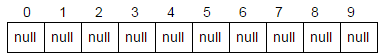ArrayList custom implementation in java/ Create own ArrayList with full code

4 >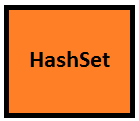Set Custom implementation in java

5 >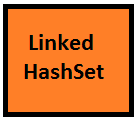LinkedHashSet Custom implementation in java

6 >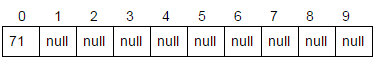Vector custom implementation in java

HashMap and Hashtable - Similarity and Differences